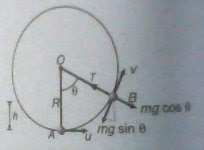# Conditions for vertical circular motion

If a bob attached to a string is swinging in vertical circular motion, we say that the bob leaves the circular path when the tension in the string becomes zero, but even when tension becomes zero, there's always a component of the bob's weight towards the center of the circle. Why then does the bob leave the circular trajectory?

bob swinging http://qph.is.quoracdn.net/main-qimg-e8a621fe0c3268ee7a2843b3ab2fc1c6?convert_to_webp=true

What is the configuration needed for a circular-motion?

The answer is, there must be an inward(towards the center) force perpendicular to the instantaneous velocity. The minimum velocity required for the bob initially to loop the whole loop is $\sqrt{5gR}$. In this situation, the tension at the topmost point becomes zero, but since there is velocity, which is $\sqrt{Rg}$ & there is inward force, which is the weight $mg$; this matches up the configuration of circular-motion & hence continues to complete the circle.

Now, if the initial velocity is less than $\sqrt{5gR}$, then the bob will have attained zero velocity before reaching the top. Now, using this velocity, we can find $h_1 \; , \; h_2$, the heights where the tension becomes zero & the height where the tangential instantaneous velocity of the bob becomes zero respectively. Using kinematics equation, we find $$h_1 = \frac{v_\text{initial}^2 + Rg}{3g} \\ h_2 = \frac{v_\text{initial}^2}{2g}$$.

Now, the case you mentioned can be described as $h_1 \lt h_2$ i.e. the tension becomes zero before velocity becomes & this implies certain velocity does exist when tension becomes zero.

The gravitational force never does point inwards i.e. towards the center of the circular trajectory. The component $mg\sin \theta$ opposes the tangential velocity & remains collinear with the instantaneous velocity vector but in the opposite direction; $mg\cos \theta$, on the other hand, points outward i.e. away from the center just opposite to the centripetal force i.e. tension. You can see in the pic$^1$ how the components of gravitational force are specified; no one is acting inwards i.e. towards the center of the circle.When tension becomes zero, velocity still exists & $mg\cos\theta$ remains perpendicular to it & is pointing inward to some point; hence it matches the configuration of circular-motion. The bob thus breaks its old circular trajectory & undergoes circular motion around a new loop where the centripetal force is $mg\cos\theta$. But it can't undergo a pure circular motion as the bob is retarded by the opposing force $mg\sin\theta$ & thus it follows a parabolic trajectory.

So, in a nut-shell, it is gravity which lets the bob to move away from the trajectory when tension becomes zero.

$^1$ Courtsey: Understanding Physics vol.I by D.C.Pandey.

Once the constraining force is broken normal parabolic motion takes over in the direction perpendicular to the tension force to the center of the circle. Depending on the angular velocity at that moment will give you the shape of parabola and mg straight downwards of the ball will be a factor. Draw a force diagram before and after the moment of release. (Rhett Alain is good for this kind of kinetic motion)